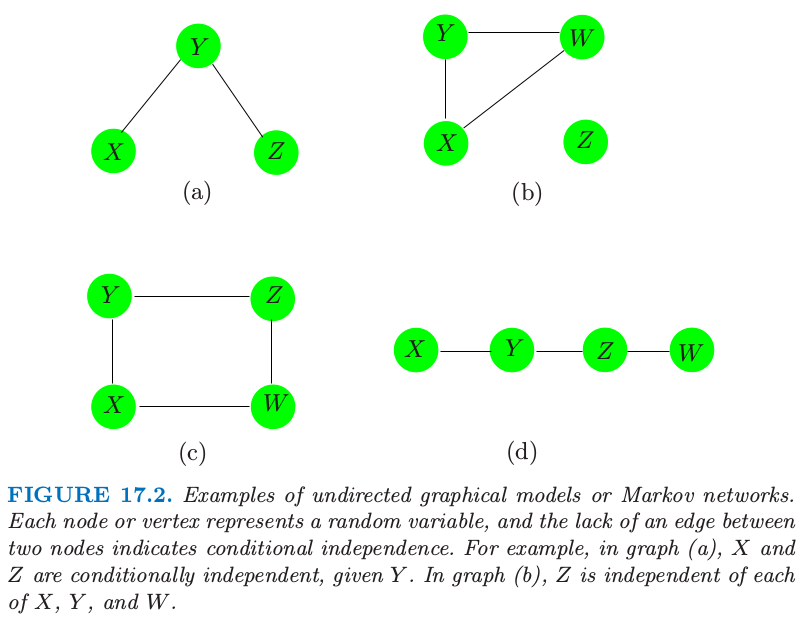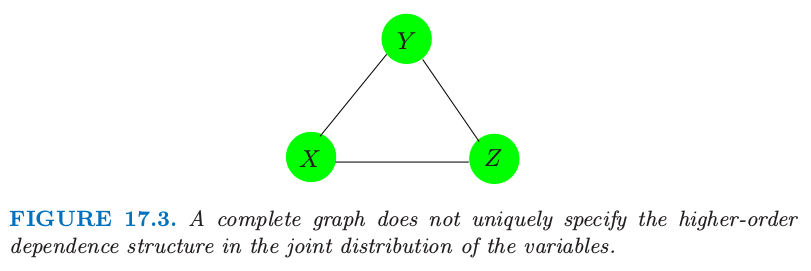17.2 马尔科夫图及其性质¶weiya 注：三种等价的 Markov 性质

pairwise Markov properties: 对于所有的非邻接顶点 $i$ 和 $j$，$a$ 为剩余结点的集合，则 $X_i\ind X_j\mid X_a$，

global Markov properties: 对于所有的不相交的子集 $a$, $b$ 和 $c$，若 $a$ 分离 $b$ 和 $c$，则 $X_b\ind X_c\mid X_a$，

local Markov properties: 对于每个顶点 $i$，$a=\mathrm{bd}(i)$ 是边界集，$b$ 为剩余结点的集合，则 $X_i\ind X_b\mid X_a$．

• $(a)\; \{X,Y\},\{Y,Z\}$
• $(b)\; \{X,Y,W\},\{Z\}$
• $(c)\; \{X,Y\},\{Y,Z\},\{Z,W\},\{X,W\}$
• $(d)\; \{X,Y\},\{Y,Z\},\{Z,W\}$

weiya 注

weiya 注：junction tree

Søren Højsgaard 的 Notes: Graphical Models with R 中通过一个简单的三结点的有向图展示了如何通过 Message Passing in Junction Tree 计算边缘分布的概率．Søren Højsgaard et al. 的 Graphical Models with R 介绍了更一般的 Message Passing in Junction Tree，主要分为两个过程，work inwards towards root 和 work outwards from root．另外，可以参考 Mark A. Paskin 的 A Short Course on Graphical Models1. Hammersley, J. M. and Clifford, P. (1971). Markov field on finite graphs and lattices, unpublished.

2. Clifford, P. (1990). Markov random fields in statistics, in G. R. Grimmett and D. J. A. Welsh (eds), Disorder in Physical Systems. A Volume in Honour of John M. Hammersley, Clarendon Press, Oxford, pp. 19–32.

3. Pearl, J. (1986). On evidential reasoning in a hierarchy of hypotheses, Artificial Intelligence 28: 9–15.

4. Lauritzen, S. and Spiegelhalter, D. (1988). Local computations with proba- bilities on graphical structures and their application to expert systems, J. Royal Statistical Society B. 50: 157–224.

5. Pearl, J. (1988). Probabilistic reasoning in intelligent systems: networks of plausible inference, Morgan Kaufmann, San Francisco, CA.

6. Shenoy, P. and Shafer, G. (1988). An axiomatic framework for Bayesian and belief-function propagation, AAAI Workshop on Uncertainty in AI, North-Holland, pp. 307–314.

7. Jensen, F. V., Lauritzen, S. and Olesen, K. G. (1990). Bayesian updating in recursive graphical models by local computation, Computational Statistics Quarterly 4: 269–282.

8. Koller, D. and Friedman, N. (2007). Structured Probabilistic Models, Stanford Bookstore Custom Publishing. (Unpublished Draft).

9. Bishop, Y., Fienberg, S. and Holland, P. (1975). Discrete Multivariate Analysis, MIT Press, Cambridge, MA. 下载